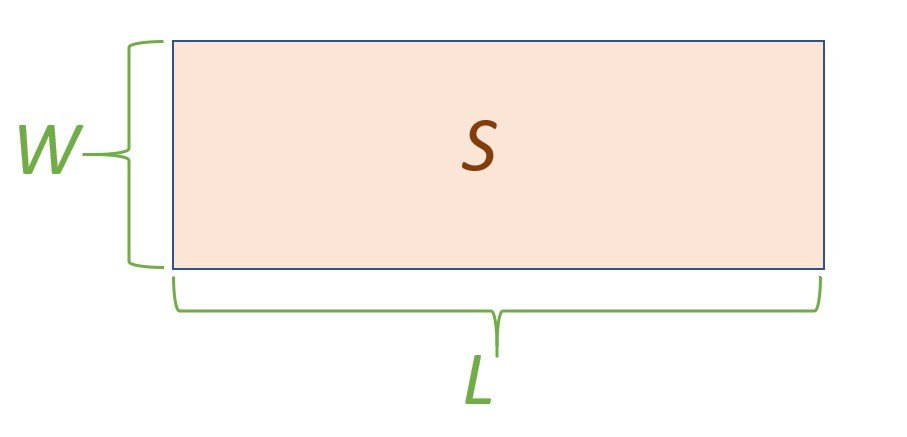# Linear meters to Square meters – lm to sqm – lin.m to m2

Enter the length in linear meters (lin. m, lm):

Enter the width of the linear meter in m (meters):

Result:

0.00m2

## Calculator reference

The figure below shows the material measured in linear meters:Legend: L – length in linear meters, W – width of a linear meter, S – area.

## How to convert a linear meter to a square meter?

You need to multiply the length in linear meters by the width of the linear meter.

A square meter is determined by the formula:

S = L * W

S is the area in square meters (sqm, m2);

L is the length in linear meters (lin. m, lm);

W is the width of a linear meter (m).

Figure with legend is shown above.

### For example:

Find the area of the carpet in square meters. The length of ​​the carpet in linear meters is 5 lm, the width of the carpet is 3 m.

S = L * W = 5 * 3 = 15 m2